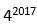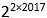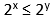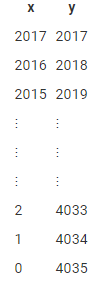# How many pairs (a, b) of positive integers - Quantitative Aptitude - Equation

## CAT 2020 - Slot 3 - Quantitative Aptitude - Equation - Question 26 - How many pairs (a, b) of positive integers

Q. 26: How many pairs (a,b) of positive integers are there such that a ≤ b and ab =?

##### 1. 20192. 20183. 20204. 2017

26. B.Number of factors of= 2×2017 + 1= 4035

Number of pairs of a,b such that a×b =is equal to half the Number of factors ofNumber of pairs of a,b such that a×b == 4035/2

You observe that 4035/2 is not an integer, because 22×2017 is a perfect square and one of the pairs of (a,b) is (2017 , 2017).

So, the number of such pairs is the highest integer roundoff of 4035/2 = 2018.

Among all these 2018 pairs, one of the integer is less than or equal to the other. (Equal in the case of (2017,2017))

We assume that a is the least one of the two…

Hence there are 2018 pairs that satify this condition.

Alternate Method:

a × b =a × b =a and b are of the form 2x and 2y respectfully…

Since a ≤ b;x ≤ y

Also, a × b =2x + 2y =x + y = 2×2017

We have 2 conditions to deal with…

x ≤ y and x + y = 2×2017

Here, x = y = 2017

From here on, we decrement x and increment y to maintain the conditions x ≤ y and x + y = 2×2017

We can keep doing this until x = 0, because if x is negative, a which is 2x will not remain an integer.Hence x can range from 2017 to 0; and thereby x can take 2018 values.

Therefore, there can be 2018 pairs of (a,b) that sitisfy a ≤ b and ab =### Past Year Question Paper & SolutionsCounselling Session
By IIM Mentor

#### Free Material Area